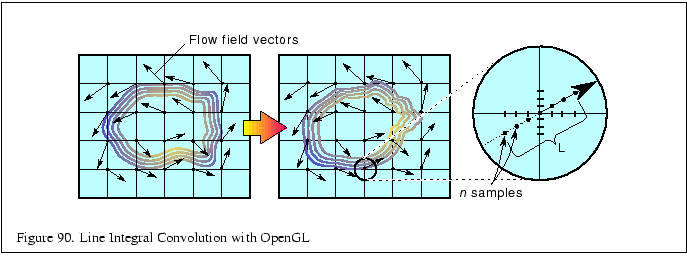Next: 16.4.3 Line Integral Convolution Up: 16.4 Line Integral Convolution Previous: 16.4.1 Sampling   Contents

### 16.4.2 Using OpenGL to Create Line Integral Convolution (LIC) Images

Instead of generating a series of custom convolution kernels and applying them to an image, you can use a texture mapping approach. This variant has the advantage that it's reasonably easy to implement and runs quickly, especially on systems with good texturing and accumulation buffer support, since it is parallelizing the convolution operations.

The concept is simple; a surface, tessellated into a mesh, is textured with an image to be processed. Each vertex on the surface has a texture coordinate associated with it. Instead of convolving the image with a series of streamline convolution kernels, the texture coordinates at each vertex are shifted parallel to flow field vector local to that vertex. This process, called advection, is done repeatedly in a series of displacements parallel to the flow vectors, with the resulting series distorted images combined using the accumulation buffer.The texture coordinates at each grid location are displaced parallel to the local field vector in a fixed series of steps. The displacement is done both parallel and antiparallel to the field vector at the vertex. The amount of displacement for each step and the number of steps determines the accuracy and appearance of the line integral convolution. The application generally sets a global value describing the length of the displacement range for all of the texture coordinates on the surface; the number of displacements along that length is computed per vertex, as a function of the local field's curl.Next: 16.4.3 Line Integral Convolution Up: 16.4 Line Integral Convolution Previous: 16.4.1 Sampling   Contents
2001-01-10